• ## 求导公式

千次阅读 2019-04-18 16:08:08
求导公式
导数四则运算
$(u \pm v)^{\prime}=u^{\prime} \pm v^{\prime} \quad(u v)^{\prime}=u^{\prime} v+u v^{\prime} \quad\left(\frac{u}{v}\right)^{\prime}=\frac{u^{\prime} v-u v^{\prime}}{v^{2}}$
基本求导公式
$y=C, \quad y^{\prime}=0$
$y=x, \quad y^{\prime}=1$
$y=x^{\mu}, \quad y^{\prime}=\mu x^{\mu-1}$
$y=a^{x}, \quad y^{\prime}=a^{x} \ln \alpha ; \quad y=e^{x}, \quad y^{\prime}=e^{x}$
$y=\log _{a} x, \quad y^{\prime}=\frac{1}{x \ln a} ; \quad y=\ln x, \quad y^{\prime}=\frac{1}{x}$
$y=\sin x, y^{\prime}=\cos x$
$y=\cos x, \quad y^{\prime}=-\sin x$
$y=\tan x, \quad y^{\prime}=\sec ^{2} x$
$y=\cot x, \quad y^{\prime}=-\csc ^{2} x$
$y=\arcsin x, \quad y^{\prime}=\frac{1}{\sqrt{1-x^{2}}}$
$(11) \quad y=\arccos x, \quad y^{\prime}=\frac{-1}{\sqrt{1-x^{2}}}$
$(12) \quad y=\arctan x, \quad y^{\prime}=\frac{1}{1+x^{2}}$
$(13) \quad y=\operatorname{arccot} x, \quad y^{\prime}=\frac{-1}{1+x^{2}}$
$(14) \quad y=\sec x, \quad y^{\prime}=\tan x \sec x$
$(15) \quad y=\csc x, \quad y^{\prime}=-\cot x \csc x$


展开全文• 《基本函数求导公式》由会员分享，可在线阅读，更多相关《基本函数求导公式(6页珍藏版)》请在人人文库网上搜索。1、基本初等函数求导公式 (1)(2)(3)(4)(5)(6)(7)(8)(9)(10)(11)(12)，(13)(14)(15)(16)函数的和、差、...
《基本函数求导公式》由会员分享，可在线阅读，更多相关《基本函数求导公式(6页珍藏版)》请在人人文库网上搜索。1、基本初等函数求导公式 (1)(2)(3)(4)(5)(6)(7)(8)(9)(10)(11)(12)，(13)(14)(15)(16)函数的和、差、积、商的求导法则设，都可导，则(1) (2) (是常数)(3) (4) 反函数求导法则若函数在某区间内可导、单调且，则它的反函数在对应区间内也可导，且或复合函数求导法则 设，而且及都可导，则复合函数的导数为或. 双曲函数与反双曲函数的导数. 双曲函数与反双曲函数都是初等函数，它们的导数都可以用前面的求导公式和求导法则求出 可以推出下表列出的公式： 一、一个方程的情形在第二章第六节中我们已经提出了隐函数的概念，并且指出了不经过显化直接由方程=0 (1。2、) 求它所确定的隐函数的方法。现在介绍隐函数存在定理，并根据多元复合函数的求导法来导出隐函数的导数公式.隐函数存在定理1 设函数在点的某一邻域内具有连续的偏导数，且，, ，则方程=0在点的某一邻域内恒能唯一确定一个单值连续且具有连续导数的函数，它满足条件，并有(2) 公式(2)就是隐函数的求导公式这个定理我们不证。现仅就公式(2)作如下推导。将方程(1)所确定的函数代入，得恒等式,其左端可以看作是的一个复合函数，求这个函数的全导数，由于恒等式两端求导后仍然恒等，即得由于连续，且，所以存在(x0,y0)的一个邻域，在这个邻域内，于是得如果的二阶偏导数也都连续，我们可以把等式(2)的两端看作的复合。3、函数而再一次求导，即得例1 验证方程在点(0,1)的某一邻域内能唯一确定一个单值且有连续导数、当=0时，的隐函数，并求这函数的一阶和二阶导数在=0的值。解 设，则,.因此由定理1可知，方程在点(0,1)的某邻域内能唯一确定一个单值且有连续导数、当=0时，的隐函数。下面求这函数的一阶和二阶导数=， ; =。隐函数存在定理还可以推广到多元函数.既然一个二元方程(1)可以确定一个一元隐函数，那末一个三元方程()=0 (3)就有可能确定一个二元隐函数。与定理1一样，我们同样可以由三元函数()的性质来断定由方程()=0所确定的二元函数=的存在，以及这个函数的性质。这就是下面的定理。隐函数存在定理2 设函。4、数()在点的某一邻域内具有连续的偏导数，且，则方程()=0在点的某一邻域内恒能唯一确定一个单值连续且具有连续偏导数的函数，它满足条件，并有=,=. (4)这个定理我们不证.与定理1类似，仅就公式(4)作如下推导.由于 (, )0,将上式两端分别对和求导，应用复合函数求导法则得+=0, +=0。因为连续，且,所以存在点的一个邻域，在这个邻域内0，于是得=,=。 例2 设，求解 设() =，则=2, =.应用公式(4)，得=。再一次对求偏导数，得二、方程组的情形下面我们将隐函数存在定理作另一方面的推广。我们不仅增加方程中变量的个数。而且增加方程的个数，例如，考虑方程组(5)这时，在四个变量中，一般。5、只能有两个变量独立变化，因此方程组(5)就有可能确定两个二元函数。在这种情形下，我们可以由函数、的性质来断定由方程组(5)所确定的两个二元函数的存在，以及它们的性质。我们有下面的定理。隐函数存在定理3 设函数、在点的某一邻域内具有对各个变量的连续偏导数，又，,且偏导数所组成的函数行列式(或称雅可比(Jacobi)式):=在点不等于零，则方程组，在点的某一邻域内恒能唯一确定一组单值连续且具有连续偏导数的函数，它满足条件，并有(6)这个定理我们不证. 例3 设，求,和.解 此题可直接利用公式(6)，但也可依照推导公式(6)的方法来求解。下面我们利用后一种方法来做。将所给方程的两边对求导并移项，得在的条件下，将所给方程的两边对求导，用同样方法在的条件下可得。
展开全文• 一、矩阵求导   一般来讲，我们约定x=(x1,x2,...xN)Tx=(x1,x2,...xN)Tx=(x_1,x_2,...x_N)^T，这是分母布局。... 其他的可以参考wiki：维基百科矩阵求导公式 二、几种重要的矩阵 1、梯度（Gr...
一、矩阵求导

一般来讲，我们约定x=(x1,x2,...xN)T$x=\left({x}_{1},{x}_{2},...{x}_{N}{\right)}^{T}$$x=(x_1,x_2,...x_N)^T$，这是分母布局。常见的矩阵求导方式有：向量对向量求导，标量对向量求导，向量对标量求导。

1、向量对向量求导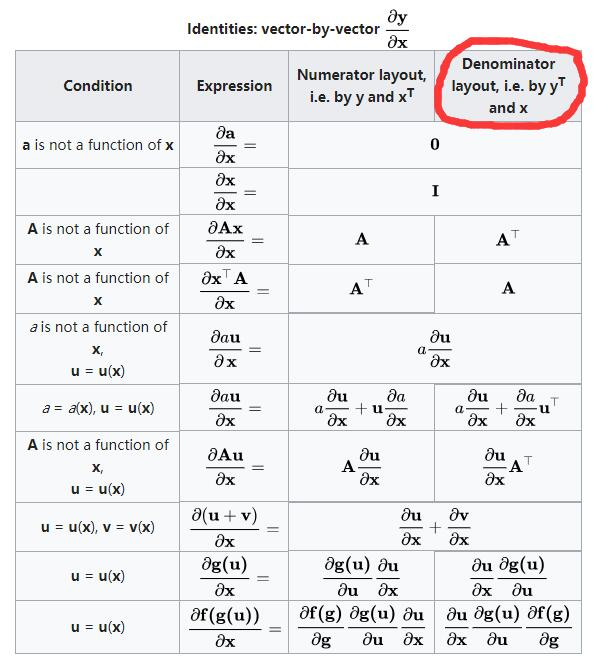2、标量对向量求导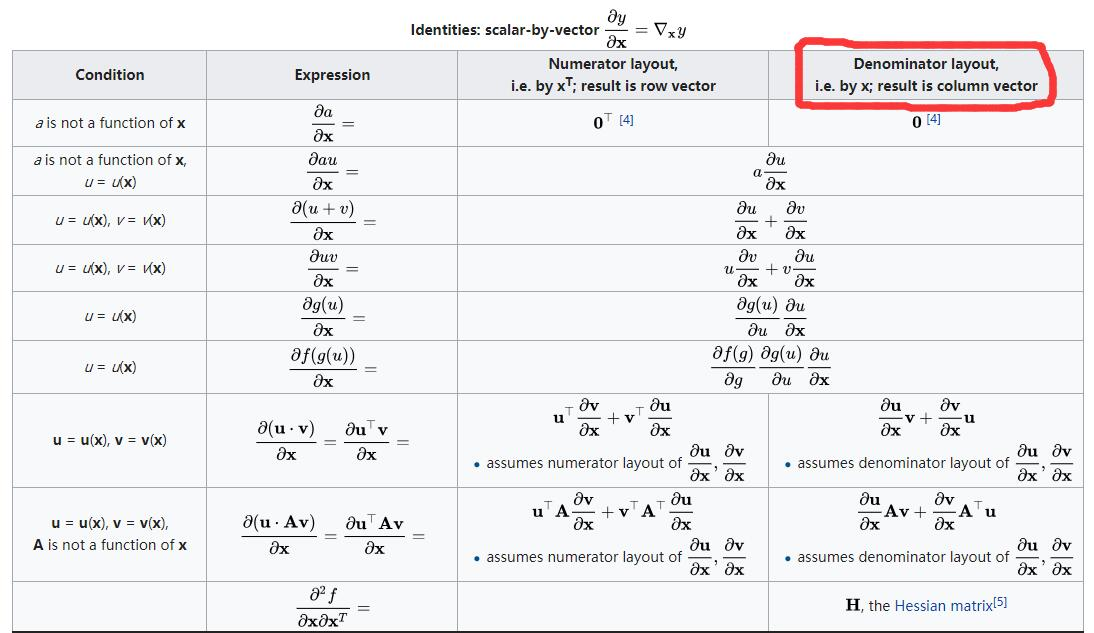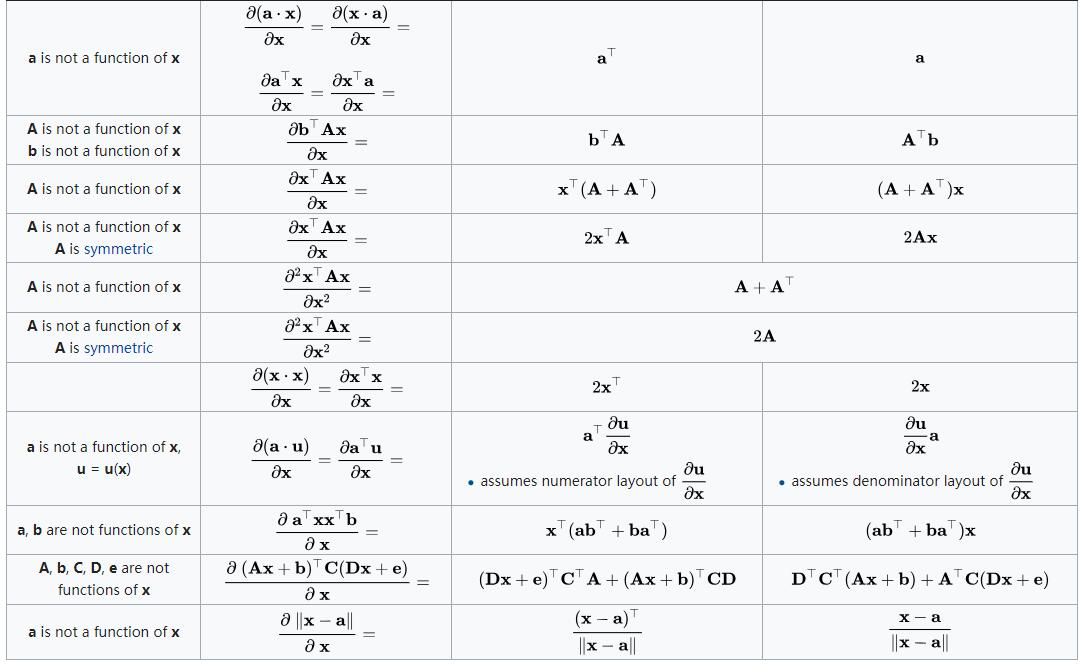3、向量对标量求导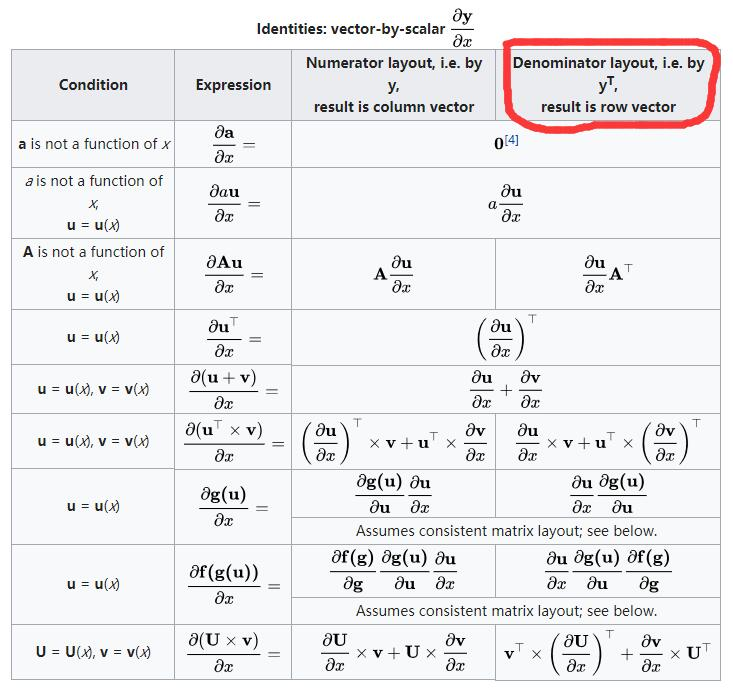其他的可以参考wiki：维基百科矩阵求导公式

二、几种重要的矩阵

1、梯度（Gradient）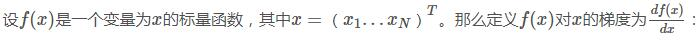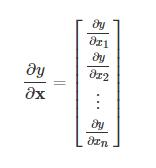2、雅克比矩阵（Jacobian matrix）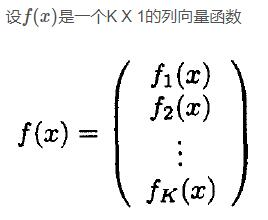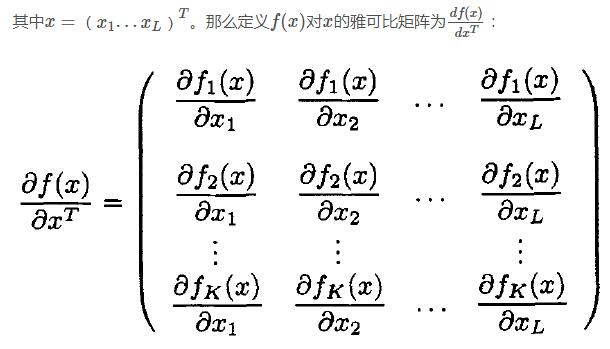3、海森矩阵（Hessian matrix）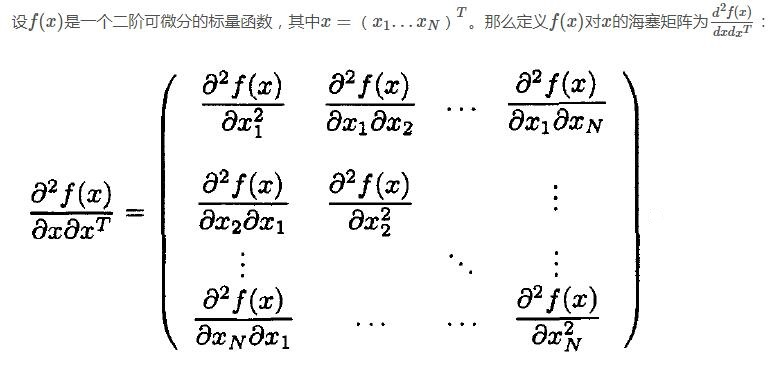三、常用的矩阵求导公式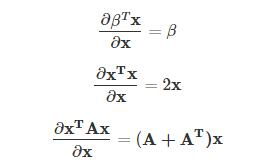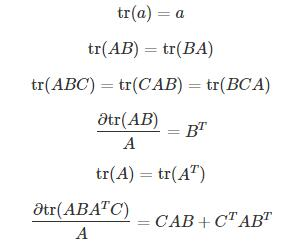参考：
https://blog.csdn.net/xtydtc/article/details/51133903
https://blog.csdn.net/yc461515457/article/details/49682473
展开全文梯度 雅克比矩阵 海森矩阵
• 大学符合函数求导公式有哪些？下文有途网小编给大家整理了复合函数的求导公式及法则，供参考！复合函数求导公式复合函数求导法则证法一：先证明个引理f(x)在点x0可导的充要条件是在x0的某邻域U(x0)内，存在一个在点...
复合函数如何求导？大学符合函数求导公式有哪些？下文有途网小编给大家整理了复合函数的求导公式及法则，供参考！复合函数求导公式复合函数求导法则证法一：先证明个引理f(x)在点x0可导的充要条件是在x0的某邻域U(x0)内，存在一个在点x0连续的函数H(x),使f(x)-f(x0)=H(x)(x-x0)从而f'(x0)=H(x0)证明：设f(x)在x0可导，令 H(x)=[f(x)-f(x0)]/(x-x0),x∈U'(x0)(x0去心邻域)；H(x)=f'(x0),x=x0因lim(x->x0)H(x)=lim(x->x0)[f(x)-f(x0)]/(x-x0)=f'(x0)=H(x0)所以H(x)在点x0连续，且f(x)-f(x0)=H(x)(x-x0),x∈U(x0)反之，设存在H(x),x∈U(x0),它在点x0连续，且f(x)-f(x0)=H(x)(x-x0),x∈U(x0)因存在极限lim(x->x0)H(x)=lim(x->x0)[f(x)-f(x0)]/(x-x0)=lim(x->x0)f'(x)=H(x0)所以f(x)在点x0可导，且f'(x0)=H(x0)引理证毕。设u=φ(x)在点u0可导，y=f(u)在点u0=φ(x0)可导，则复合函数F(x)=f(φ(x))在x0可导，且F'(x0)=f'(u0)φ'(x0)=f'(φ(x0))φ'(x0)证明：由f(u)在u0可导，由引理必要性，存在一个在点u0连续的函数H(u),使f'(u0)=H(u0),且f(u)-f(u0)=H(u)(u-u0)又由u=φ(x)在x0可导，同理存在一个在点x0连续函数G(x),使φ'(x0)=G(x0),且φ(x)-φ(x0)=G(x)(x-x0)于是就有，f(φ(x))-f(φ(x0))=H(φ(x))(φ(x)-φ(x0))=H(φ(x))G(x)(x-x0)因为φ，G在x0连续，H在u0=φ(x0)连续，因此H(φ(x))G(x)在x0连续，再由引理的充分性可知F(x)在x0可导，且F'(x0)=f'(u0)φ'(x0)=f'(φ(x0))φ'(x0)证法二：y=f(u)在点u可导，u=g(x)在点x可导，则复合函数y=f(g(x))在点x0可导，且dy/dx=(dy/du)*(du/dx)证明：因为y=f(u)在u可导，则lim(Δu->0)Δy/Δu=f'(u)或Δy/Δu=f'(u)+α(lim(Δu->0)α=0)当Δu≠0，用Δu乘等式两边得，Δy=f'(u)Δu+αΔu但当Δu=0时，Δy=f(u+Δu)-f(u)=0,故上等式还是成立。又因为Δx≠0,用Δx除以等式两边，且求Δx->0的极限，得dy/dx=lim(Δx->0)Δy/Δx=lim(Δx->0)[f'(u)Δu+αΔu]/Δx=f'(u)lim(Δx->0)Δu/Δx+lim(Δx->0)αΔu/Δx又g(x)在x处连续(因为它可导),故当Δx->0时，有Δu=g(x+Δx)-g(x)->0则lim(Δx->0)α=0最终有dy/dx=(dy/du)*(du/dx)
展开全文• 反函数求导公式2020-09-12 08:55:01文/张敏反函数的导数是原函数导数的倒数。求y=arcsinx的导函数，反函数的导数就是原函数导数的倒数。首先，函数y=arcsinx的反函数为x=siny，所以：y‘=1/sin’y=1/cosy，因为x=...
• 变限积分的求导公式及其应用周少波;雷冬霞;程生敏【期刊名称】《学园》【年(卷),期】2012(000)019【摘要】本文针对学生难以掌握的变限定积分的最为一般的求导公式，给出了学生易于理解和接受的一元函数的证明，并用...
• 复合函数求导公式有哪些2018-08-01 17:24:23文/丁雪竹有很多的同学是非常的想知道，复合函数求导公式是什么，小编整理了相关信息，希望会对大家有所帮助！复合函数如何求导规则：1、设u=g(x)，对f(u)求导得：f'(x)=f...
•数学建模
•数学
• 后期再补充求导法则与反三角函数等求导公式。完美结束如果大家看完这篇文章，能有很大的收获，我就开心啦。希望大家喜欢，更多文章敬请期待。END欢迎交流，有错误及时指出（可以直接在评论区指出，大...
• 基本初等函数求导公式与微分公式.(C ) ' 0 ( x ) ' x 1 (ax)' ax lna (e x ) ' e x dC 0 d ( x ) x 1dx d(ax) ax lnxdx d (e x ) e x dx
• 常见求导公式 (arcsinx)′=11−x2(arcsinx)'=\frac{1}{\sqrt{1-x^2}}(arcsinx)′=1−x2​1​ (arccosx)′=−11−x2(arccosx)'=-\frac{1}{\sqrt{1-x^2}}(arccosx)′=−1−x2​1​ (arctanx)′=11+x2(arctanx)'=\frac{...
• ## 常用的向量矩阵求导公式

万次阅读 多人点赞 2016-10-14 14:29:06
总结下数理推导中常用的向量矩阵求导公式，方便以后查询。矩阵
• 展开全部类型1、下限为常数，上限为函数类型第一步：对于这种类型只需将62616964757a686964616fe4b893e5b19e31333365666262上限函数代入到积分的原函数中去，再对上限函数进行求导。第二步：对下面的函数进行求导，...
• ## 向量求导公式

千次阅读 2016-12-15 16:00:01
最近在看吴恩达的视频啊、LDA啊、PCA啊，觉得很有必要将向量求导公式复习一下，要不感觉算的时候怪怪的~...